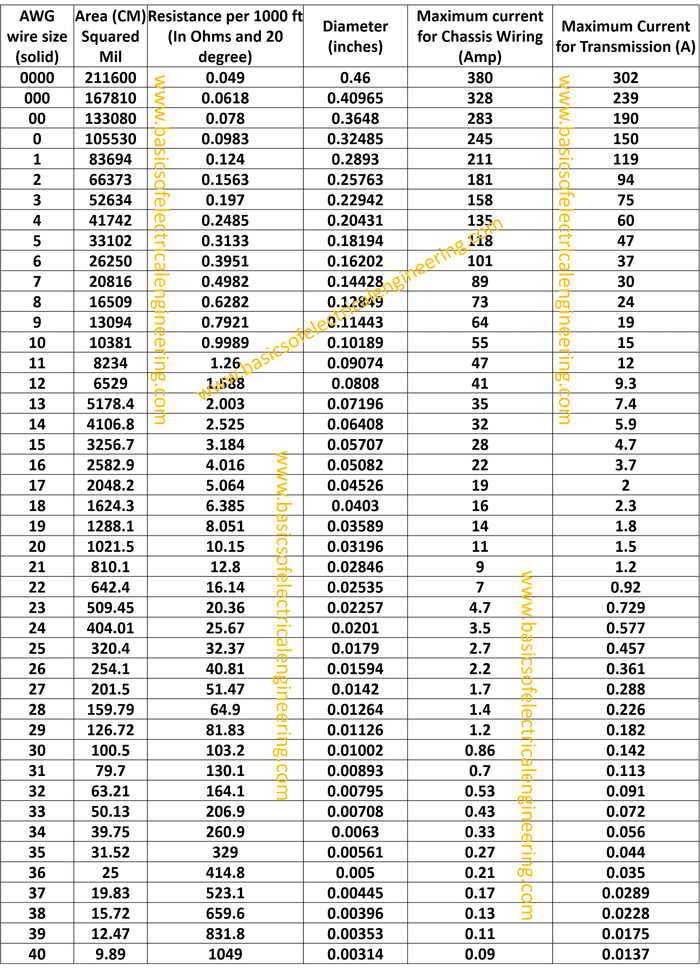# Basics of Electrical Engineering

Learn the basics of Electrical Engineering.

We previously studied about current. But how to determine the diameter of wire for carrying a specific amount of current.

Wire gauge is the measurement of wire diameter. Wire gauge actually explains how much electrical current a wire can safely carry. American Wire Gauge or AWG is a wire table which is the primary system used for selecting wires. Let's have a look at it:Here a few points for using the table.
1. The table is for solid wires. Practically, the wires are stranded (Made of many strands instead of a single solid conductor.
2. For a change of gauge number by 3 the area is doubled (approx). That is for AWG 37 the area is 19.83 whereas for 34 it is 39.76 which is approximately double in number.

#### 2 comments:

1.Thanks for sharing, this is a fantastic article. Will read on… electricians gulfport ms

2.Since when wires are exposed to vibrations, stress, climate, and different things that are continually present in vehicles, they will in general erode. At the point when wires erode, you get... soow cable